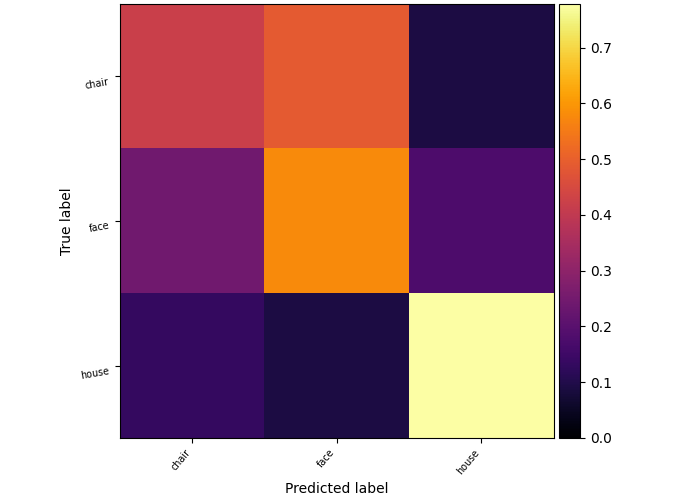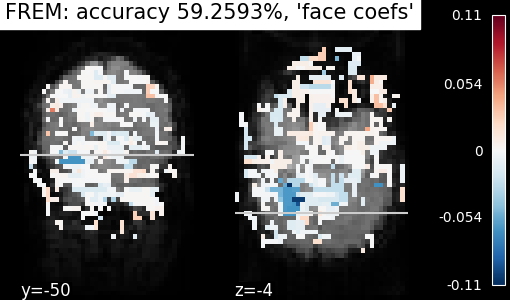# Decoding with FREM: face vs house vs chair object recognition#

This example uses fast ensembling of regularized models (FREM) to decode a face vs house vs chair discrimination task from Haxby 2001 study. FREM uses an implicit spatial regularization through fast clustering and aggregates a high number of estimators trained on various splits of the training set, thus returning a very robust decoder at a lower computational cost than other spatially regularized methods.

To have more details, see: FREM: fast ensembling of regularized models for robust decoding.

```from nilearn.datasets import fetch_haxby

data_files = fetch_haxby()

import pandas as pd

# Restrict to face, house, and chair conditions
conditions = behavioral["labels"]

# Split data into train and test samples, using the chunks

# Apply this sample mask to X (fMRI data) and y (behavioral labels)
# Because the data is in one single large 4D image, we need to use
# index_img to do the split easily
from nilearn.image import index_img

func_filenames = data_files.func

# Compute the mean epi to be used for the background of the plotting
from nilearn.image import mean_img

background_img = mean_img(func_filenames)
```

## Fit FREM#

```from nilearn.decoding import FREMClassifier

decoder = FREMClassifier(cv=10, standardize="zscore_sample")
# Fit model on train data and predict on test data
decoder.fit(X_train, y_train)
y_pred = decoder.predict(X_test)
accuracy = (y_pred == y_test).mean() * 100.0
print(f"FREM classification accuracy : {accuracy:g}%")
```
```/home/remi/github/nilearn/env/lib/python3.11/site-packages/nilearn/_utils/param_validation.py:213: UserWarning:

Brain mask is bigger than the volume of a standard human brain. This object is probably not tuned to be used on such data.

/home/remi/github/nilearn/env/lib/python3.11/site-packages/sklearn/feature_selection/_univariate_selection.py:112: UserWarning:

Features  are constant.

/home/remi/github/nilearn/env/lib/python3.11/site-packages/sklearn/feature_selection/_univariate_selection.py:113: RuntimeWarning:

invalid value encountered in divide

/home/remi/github/nilearn/env/lib/python3.11/site-packages/sklearn/feature_selection/_univariate_selection.py:113: RuntimeWarning:

divide by zero encountered in divide

/home/remi/github/nilearn/env/lib/python3.11/site-packages/sklearn/feature_selection/_univariate_selection.py:112: UserWarning:

Features  are constant.

/home/remi/github/nilearn/env/lib/python3.11/site-packages/sklearn/feature_selection/_univariate_selection.py:113: RuntimeWarning:

invalid value encountered in divide

/home/remi/github/nilearn/env/lib/python3.11/site-packages/sklearn/feature_selection/_univariate_selection.py:113: RuntimeWarning:

divide by zero encountered in divide

/home/remi/github/nilearn/env/lib/python3.11/site-packages/sklearn/feature_selection/_univariate_selection.py:112: UserWarning:

Features  are constant.

/home/remi/github/nilearn/env/lib/python3.11/site-packages/sklearn/feature_selection/_univariate_selection.py:113: RuntimeWarning:

invalid value encountered in divide

/home/remi/github/nilearn/env/lib/python3.11/site-packages/sklearn/feature_selection/_univariate_selection.py:113: RuntimeWarning:

divide by zero encountered in divide

FREM classification accuracy : 57.7778%
```

## Plot confusion matrix#

```import numpy as np
from sklearn.metrics import confusion_matrix

from nilearn import plotting

# Calculate the confusion matrix
matrix = confusion_matrix(
y_test,
y_pred,
normalize="true",
)

# Plot the confusion matrix
im = plotting.plot_matrix(
matrix,
labels=sorted(np.unique(y_test)),
vmin=0,
cmap="hot_r",
)

ax = im.axes
ax.set_ylabel("True label")
ax.set_xlabel("Predicted label")

# Adjust figure to make labels fit
ax.get_figure().tight_layout()

plotting.show()
```## Visualization of FREM weights#

```from nilearn import plotting

plotting.plot_stat_map(
decoder.coef_img_["face"],
background_img,
title=f"FREM: accuracy {accuracy:g}%, 'face coefs'",
cut_coords=(-50, -4),
display_mode="yz",
)
plotting.show()
```FREM ensembling procedure yields an important improvement of decoding accuracy on this simple example compared to fitting only one model per fold and the clustering mechanism keeps its computational cost reasonable even on heavier examples. Here we ensembled several instances of l2-SVC, but FREMClassifier also works with ridge or logistic. FREMRegressor object is also available to solve regression problems.

Total running time of the script: (7 minutes 47.415 seconds)

Estimated memory usage: 2017 MB

Gallery generated by Sphinx-Gallery